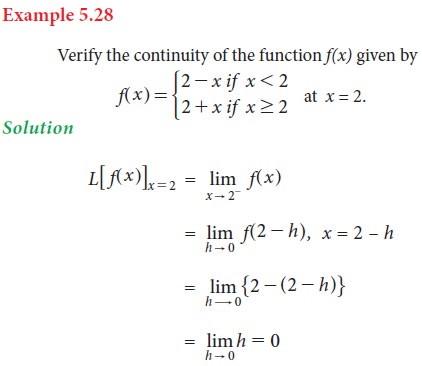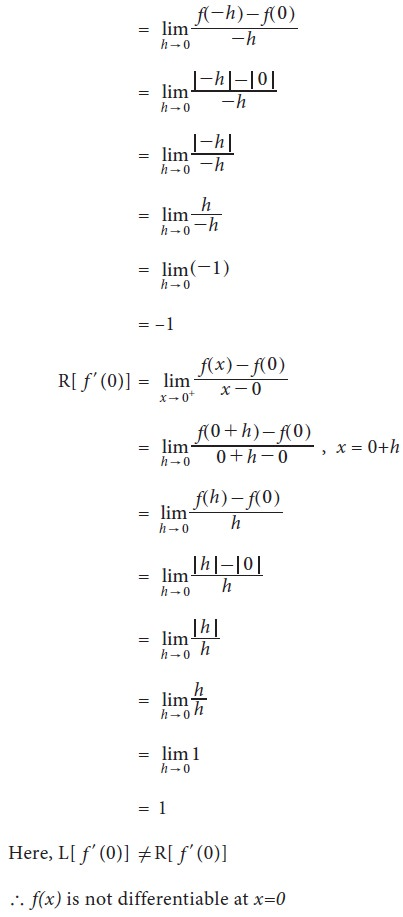Home | | Business Maths 11th std | Limits and derivatives

# Limits and derivatives

Limits are used when we have to find the value of a function near to some value.

Limits and derivatives

In this section, we will discuss about limits, continuity of a function, differentiability and differentiation from first principle. Limits are used when we have to find the value of a function near to some value.## 1. Existence of limit## 2. Algorithm of left hand limit : L[f(x)]x=a## 3. Algorithm of right hand limit : R[f(x)]x=a## 4. Some results of limits## 5. Indeterminate forms and evaluation of limits## 6. Methods of evaluation of algebraic limits

1.     Direct substitution

2.     Factorization

3.     Rationalisation

4.     By using some standard limits

## 7. Some standard limits### Derivative

Before going into the topic, let us first discuss about the continuity of a function. A function f(x) is continues at x = a if its graph has no break at x = a

If there is any break at the point x = a then we say that the function is not continuous at the point x = a.

If a function is continuous at all the point in an interval, then it is said to be a continuous in that interval.

## 8. Continuous function## 9. Some properties of continuous functions## 10. Differentiability at a point## 11. Left hand derivative and right hand derivative## 12. Differentiation from first principle## 13. Derivatives of some standard functions using first principleTags : Definition, Formula, Solved Example Problems, Exercise | Differential Calculus | Mathematics , 11th Business Mathematics and Statistics(EMS) : Chapter 5 : Differential Calculus
Study Material, Lecturing Notes, Assignment, Reference, Wiki description explanation, brief detail
11th Business Mathematics and Statistics(EMS) : Chapter 5 : Differential Calculus : Limits and derivatives | Definition, Formula, Solved Example Problems, Exercise | Differential Calculus | Mathematics